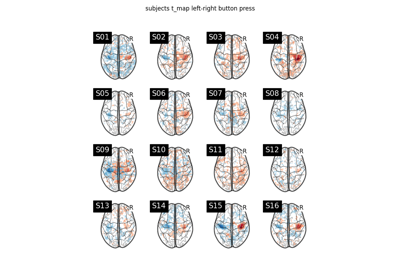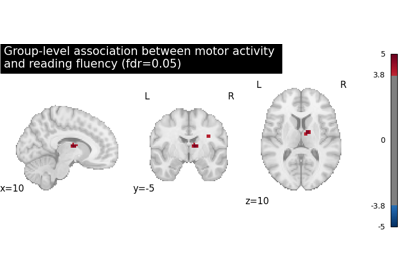Note

This page is a reference documentation. It only explains the function signature, and not how to use it. Please refer to the user guide for the big picture.

# nilearn.glm.second_level.non_parametric_inference#

nilearn.glm.second_level.non_parametric_inference(second_level_input, confounds=None, design_matrix=None, second_level_contrast=None, first_level_contrast=None, mask=None, smoothing_fwhm=None, model_intercept=True, n_perm=10000, two_sided_test=False, random_state=None, n_jobs=1, verbose=0, threshold=None, tfce=False)[source]#

Generate p-values corresponding to the contrasts provided based on permutation testing.

This function is a light wrapper around `permuted_ols`, with additional steps to ensure compatibility with the `second_level` module.

Parameters:
second_level_input`list` of `FirstLevelModel` objects or `pandas.DataFrame` or `list` of Niimg-like objects.
• Giving `FirstLevelModel` objects will allow to easily compute the second level contrast of arbitrary first level contrasts thanks to the first_level_contrast argument of `compute_contrast`. Effect size images will be computed for each model to contrast at the second level.

• If a `DataFrame`, then it has to contain subject_label, map_name and effects_map_path. It can contain multiple maps that would be selected during contrast estimation with the argument first_level_contrast of `compute_contrast`. The `DataFrame` will be sorted based on the subject_label column to avoid order inconsistencies when extracting the maps. So the rows of the automatically computed design matrix, if not provided, will correspond to the sorted subject_label column.

• If a `list` of Niimg-like objects then this is taken literally as Y for the model fit and design_matrix must be provided.

confounds`pandas.DataFrame` or None, optional

Must contain a subject_label column. All other columns are considered as confounds and included in the model. If `design_matrix` is provided then this argument is ignored. The resulting second level design matrix uses the same column names as in the given `DataFrame` for confounds. At least two columns are expected, `subject_label` and at least one confound.

design_matrix`pandas.DataFrame` or None, optional

Design matrix to fit the GLM. The number of rows in the design matrix must agree with the number of maps derived from `second_level_input`. Ensure that the order of maps given by a `second_level_input` list of Niimgs matches the order of the rows in the design matrix.

second_level_contrast`str` or `numpy.ndarray` of shape(n_col), optional

Where n_col is the number of columns of the design matrix. The string can be a formula compatible with `pandas.DataFrame.eval`. Basically one can use the name of the conditions as they appear in the design matrix of the fitted model combined with operators +- and combined with numbers with operators +-*/. The default None is accepted if the design matrix has a single column, in which case the only possible contrast array((1)) is applied; when the design matrix has multiple columns, an error is raised.

first_level_contrast`str`, optional

In case a pandas DataFrame was provided as second_level_input this is the map name to extract from the pandas dataframe map_name column. It has to be a ‘t’ contrast.

New in version 0.9.0.

maskNiimg-like, `NiftiMasker` or `MultiNiftiMasker` object, optional

Mask to be used on data. If an instance of masker is passed, then its mask will be used. If no mask is given, it will be computed automatically by a `MultiNiftiMasker` with default parameters. Automatic mask computation assumes first level imgs have already been masked.

smoothing_fwhm`float`, optional.

If smoothing_fwhm is not None, it gives the full-width at half maximum in millimeters of the spatial smoothing to apply to the signal.

model_intercept`bool`, optional

If `True`, a constant column is added to the confounding variates unless the tested variate is already the intercept. Default=True.

n_perm`int`, optional

Number of permutations to perform. Permutations are costly but the more are performed, the more precision one gets in the p-values estimation. Default=10000.

two_sided_test`bool`, optional
• If `True`, performs an unsigned t-test. Both positive and negative effects are considered; the null hypothesis is that the effect is zero.

• If `False`, only positive effects are considered as relevant. The null hypothesis is that the effect is zero or negative.

Default=False.

random_state`int` or RandomState, optional

Pseudo-random number generator state used for random sampling. Use this parameter to have the same permutations in each computing units.

n_jobs`int`, default=1

The number of CPUs to use to do the computation. -1 means ‘all CPUs’.

verbose`int`, default=0

Verbosity level (0 means no message).

thresholdNone or `float`, optional

Cluster-forming threshold in p-scale. This is only used for cluster-level inference. If None, no cluster-level inference will be performed. Default=None.

Warning

Performing cluster-level inference will increase the computation time of the permutation procedure.

New in version 0.9.2.

tfce`bool`, optional

Whether to calculate TFCE as part of the permutation procedure or not. The TFCE calculation is implemented as described in Smith and Nichols. Default=False.

Warning

Performing TFCE-based inference will increase the computation time of the permutation procedure considerably. The permutations may take multiple hours, depending on how many permutations are requested and how many jobs are performed in parallel.

New in version 0.9.2.

Returns:
neg_log10_vfwe_pvals_img`Nifti1Image`

The image which contains negative logarithm of the voxel-level FWER-corrected p-values.

Note

This is returned if `threshold` is None (the default).

outputs`dict`

Output images, organized in a dictionary. Each image is 3D/4D, with the potential fourth dimension corresponding to the regressors.

Note

This is returned if `tfce` is False or `threshold` is not None.

New in version 0.9.2.

Here are the keys:

key

description

t

T-statistics associated with the significance test of the n_regressors explanatory variates against the n_descriptors target variates.

logp_max_t

Negative log10 family-wise error rate-corrected p-values corrected based on the distribution of maximum t-statistics from permutations.

size

Cluster size values associated with the significance test of the n_regressors explanatory variates against the n_descriptors target variates.

Returned only if `threshold` is not None.

logp_max_size

Negative log10 family-wise error rate-corrected p-values corrected based on the distribution of maximum cluster sizes from permutations. This map is generated through cluster-level methods, so the values in the map describe the significance of clusters, rather than individual voxels.

Returned only if `threshold` is not None.

mass

Cluster mass values associated with the significance test of the n_regressors explanatory variates against the n_descriptors target variates.

Returned only if `threshold` is not None.

logp_max_mass

Negative log10 family-wise error rate-corrected p-values corrected based on the distribution of maximum cluster masses from permutations. This map is generated through cluster-level methods, so the values in the map describe the significance of clusters, rather than individual voxels.

Returned only if `threshold` is not None.

tfce

TFCE values associated with the significance test of the n_regressors explanatory variates against the n_descriptors target variates.

Returned only if `tfce` is True.

logp_max_tfce

Negative log10 family-wise error rate-corrected p-values corrected based on the distribution of maximum TFCE values from permutations.

Returned only if `tfce` is True.

`permuted_ols`

References

## Examples using `nilearn.glm.second_level.non_parametric_inference`#Second-level fMRI model: one sample test

Second-level fMRI model: one sample test